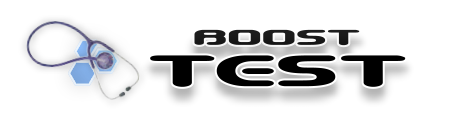> The Unit Test Framework > Testing tools > Floating-point comparison algorithms

#### Floating-point comparison algorithms

In most cases it is unreasonable to use an `operator==(...)` for a floating-point values equality check. The simple, absolute value comparison based, solution for a floating-point values `u`, `v` and a tolerance :

 |`u` `v`| (1)

does not produce expected results in many circumstances - specifically for very small or very big values (See [Squassabia] for examples). The UTF implements floating-point comparison algorithm that is based on the more confident solution first presented in [KnuthII]:

 |`u` `v`| |`u`| |`u` `v`| |`v`| (2)

defines a very close with tolerance relationship between `u` and `v`

 |`u` `v`| |`u`| |`u` `v`| |`v`| (3)

defines a close enough with tolerance relationship between `u` and `v`

Both relationships are commutative but are not transitive. The relationship defined by inequations (2) is stronger that the relationship defined by inequations (3) (i.e. (2) (3)). Because of the multiplication in the right side of inequations, that can cause an unwanted underflow condition, the implementation is using modified version of the inequations (2) and (3) where all underflow, overflow conditions can be guarded safely:

 |`u` `v`| |`u`| |`u` `v`| / |`v`| (4)
 |`u` `v`| |`u`| |`u` `v`| / |`v`| (5)

Checks based on equations (4) and (5) are implemented by two predicates with alternative interfaces: binary predicate `close_at_tolerance` and predicate with four arguments `check_is_close`.

While equations (4) and (5) in general are preferred for the general floating point comparison check over equation (1), they are unusable for the test on closeness to zero. The later check is still might be useful in some cases and the UTF implements an algorithm based on equation (1) in binary predicate `check_is_small`.

On top of the generic, flexible predicates the UTF implements macro based family of tools `BOOST_CHECK_CLOSE` and `BOOST_CHECK_SMALL`. These tools limit the check flexibility to strong-only checks, but automate failed check arguments reporting.

##### Tolerance selection considerations

In case of absence of domain specific requirements the value of tolerance can be chosen as a sum of the predicted upper limits for "relative rounding errors" of compared values. The "rounding" is the operation by which a real value 'x' is represented in a floating-point format with 'p' binary digits (bits) as the floating-point value 'X'. The "relative rounding error" is the difference between the real and the floating point values in relation to real value: |x-X|/|x|. The discrepancy between real and floating point value may be caused by several reasons:

• Type promotion
• Arithmetic operations
• Conversion from a decimal presentation to a binary presentation
• Non-arithmetic operation

The first two operations proved to have a relative rounding error that does not exceed "machine epsilon value" for the appropriate floating point type (represented by `std::numeric_limits`<FPT>::epsilon()). Conversion to binary presentation, sadly, does not have such requirement. So we can't assume that float 1.1 is close to real 1.1 with tolerance "machine epsilon value" for float (though for 11./10 we can). Non arithmetic operations either do not have a predicted upper limit relative rounding errors. Note that both arithmetic and non-arithmetic operations might also produce others "non-rounding" errors, such as underflow/overflow, division-by-zero or 'operation errors'.

All theorems about the upper limit of a rounding error, including that of epsilon, refer only to the 'rounding' operation, nothing more. This means that the 'operation error', that is, the error incurred by the operation itself, besides rounding, isn't considered. In order for numerical software to be able to actually predict error bounds, the IEEE754 standard requires arithmetic operations to be 'correctly or exactly rounded'. That is, it is required that the internal computation of a given operation be such that the floating point result is the exact result rounded to the number of working bits. In other words, it is required that the computation used by the operation itself doesn't introduce any additional errors. The IEEE754 standard does not require same behavior from most non-arithmetic operation. The underflow/overflow and division-by-zero errors may cause rounding errors with unpredictable upper limits.

At last be aware that epsilon rules are not transitive. In other words combination of two arithmetic operations may produce rounding error that significantly exceeds 2 epsilon. All in all there are no generic rules on how to select the tolerance and users need to apply common sense and domain/ problem specific knowledge to decide on tolerance value.

To simplify things in most usage cases latest version of algorithm below opted to use percentage values for tolerance specification (instead of fractions of related values). In other words now you use it to check that difference between two values does not exceed x percent.

### Books

[Kulisch] Rounding near zero. Advanced Arithmetic for the Digital Computer. Copyright © 2002 Springer, Inc.. 0-201-89684-2. Springer; 1 edition.

### Periodicals

[Squassabia] C++ Report. March 2000. .

[Becker] “The Journeyman's Shop: Trap Handlers, Sticky Bits, and Floating-Point Comparisons”. C/C++ Users Journal. December 2000. .

### Publications

[Goldberg] What Every Computer Scientist Should Know About Floating-Point Arithmetic”. Copyright © 1991 Association for Computing Machinery, Inc.. 150-230. Computing Surveys. March. .

 `v` is zero